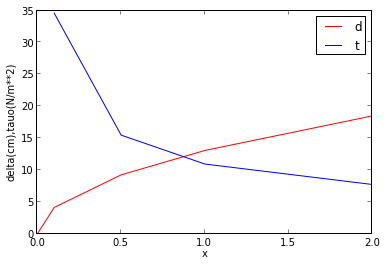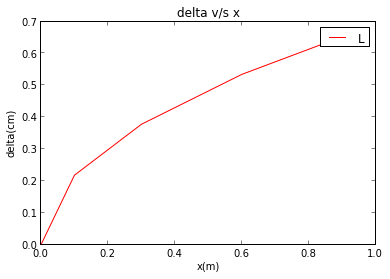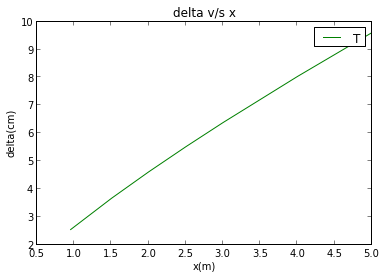## Chapter 7 : Fluid Resistance¶

### Example 7.1 Page no 245¶

In :
# Is the flow laminar or turbulent

from math import *

from __future__ import division

# Given

nu1 = 0.804*10**-6               # viscosity in m**2/s

V = 0.3                        # velocity in m/s

D = 0.02                       # diameter in m/s

# for water

rho = 995.7                    # density in kg/m**3

# for gylcerine

mu = 8620*10**-4               # viscosity in Ns/m**2

S = 1.26                       # specific gravity

nu2 = mu/(S*rho)                # viscosity of glycerine in Ns/m**2

# Solution

R1 = V*D/nu1

print "Reynolds number for water =",round(R1,0)

print "R > 2000 the flow is turbulent for water"

print "\n"
R2 = V*D/nu2

print "Reynolds number for glycerine =",round(R2,1)

print "R < 2000 the flow is laminar for glycerine"

Reynolds number for water = 7463.0
R > 2000 the flow is turbulent for water

Reynolds number for glycerine = 8.7
R < 2000 the flow is laminar for glycerine


### Example 7.2 Page no 248¶

In :
# Eddy Viscosity; mixing length ; turbulence constant

from math import *

from __future__ import division

from scipy import *

import numpy as np

from sympy import *

y = Symbol('y')

d = 0.0175           # diameter in m

s = 0.3              # shear stress at a distance in m

tau = 103            # shear stress in Pa

rho = 1000           # density in kg/m**3

#y = 0.3

# solution

Up = diff(8.5+0.7*log(y),y)

print Up

Up = (0.7/0.3)                     # for y = 0.3

k = sqrt(tau/(rho*s**2*Up**2))

print "Turbulence constant = ",round(k,2)

Ml = k*s*100             # mixing length

print "Mixing length = ",round(Ml,1),"cm"

Eta = rho*(Ml/100)**2*Up

print "Eddy viscosity =",round(Eta,1),"Nm/s**2"

0.7/y
Turbulence constant =  0.46
Mixing length =  13.8 cm
Eddy viscosity = 44.1 Nm/s**2


### Example 7.3 Page no 256¶

In :
# PLot boundary layer distribution and total drag on the plate

from math import *

from __future__ import division

from pylab import plt

from numpy import *

from scipy import *

from sympy import *

import matplotlib.pyplot as plt

# Given

# for glycerine

S = 1.26                  # specific gravity

mu = 0.862                # dynamic viscosity in Ns/m**2

rho = S *1000             # density in kg/m**3

K2 = 0.332

V=1                       # velocity in m/s

# Solution

# from blasius equation

x = [0,0.1,0.5,1.0,2.0];

d = 0.1307*np.sqrt(x)*100

tauo = K2*rho*V**2/(sqrt(1462)*np.sqrt(x))

#plt.figure()
plt.plot(x, d, 'r')
plt.xlabel('x(m)')
plt.ylabel('delta(cm),tauo(N/m**2)')
#plt.title('delta v/s x')
#plt.legend('d')

plt.plot(x, tauo, 'b')
plt.xlabel('x')
#plt.ylabel('tauo(N/m**2)')
#plt.title('tauo v/s x(m)')
plt.legend('d''t')
plt.show()### Example 7.4 page no 260¶

In :
# Sketch the boundary layer and drag on the plate

import numpy as np

from math import *

from __future__ import division

import matplotlib.pyplot as plt

from numpy import sqrt

# Given

rho = 1.197               # air density in kg/m**3

mu = 18.22*10**-6         # viscosity in Ns/m**2

l = 5                     # length of the plate

V = 8                     # velocity in m/s

Rec = 5*10**5              # crictical reynolds number

l1 = 0.951                # length from 0 to 0.951

l2 = 5.0                  # length from 0 to 5

l3 = 0.951                # length from 0 to 0.951

# Solution

X = Rec/525576

x = [0,0.1,0.3,0.6,0.951];

d = 0.0069*np.sqrt(x)*100

plt.figure()
plt.plot(x, d, 'r')
plt.xlabel('x(m)')
plt.ylabel('delta(cm)')
plt.title('delta v/s x')
plt.legend('L')
plt.show()

X1 = [0.951,1.5,2.0,2.5,3.0,4.0,5.0]

Dt = 0.0265*np.power(X1,(4/5))*100

plt.figure()
plt.plot(X1, Dt, 'g')
plt.xlabel('x(m)')
plt.ylabel('delta(cm)')
plt.title('delta v/s x')
plt.legend('T')
plt.show()

Td = 0.664*sqrt(mu*rho*V**3*l1)+0.036*rho*V**2*l2*(mu/(rho*V*l2))**0.2-0.036*rho*V**2*l3*(mu/(rho*V*l3))**0.2

print "Total Drag = ",round(Td,3),"N"Total Drag =  0.595 N


### Example 7.5 Page no 270¶

In :
# Determine drag on the sphere

from math import *

from pylab import plt

from __future__ import division

# Given

d = 0.01         # doameter of sphere in m

v = 0.05         # velocity in m/s

S = 1.26         # specific gravity

mu = 0.826       # kinematic viscosity in Ns/m**2

rho = S*1000     # density

# Solution

R = rho*v*d/mu

# for the above rho

Cd = 35

Fd = 0.5*Cd*rho*v**2*pi*d**2/4

print "Drag on the sphere = ",round(Fd,4),"N"

Drag on the sphere =  0.0043 N

In [ ]: